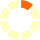Standard:

Description:

Standard:

# Math.5.NF.5b or 5.NF.B.5.B

Description:

## Explaining why multiplying a given number by a fraction greater than 1 results in a product greater than the given number (recognizing multiplication by whole numbers greater than 1 as a familiar case); explaining why multiplying a given number by a fraction less than 1 results in a product smaller than the given number; and relating the principle of fraction equivalence a/b = (n × a)/(n × b) to the effect of multiplying a/b by 1.5th Grade Math - Factors & Products Lesson# Processing Request...